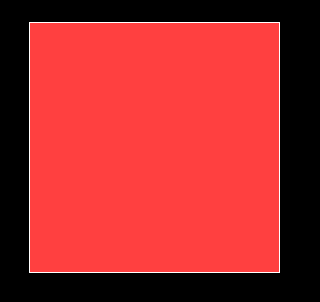# Flood fill algorithm using C graphics

Given a rectangle, your task to fill this rectangle using flood fill algorithm.
Examples:

```Input : rectangle(left = 50, top = 50, right= 100, bottom = 100)
flood( x = 55, y = 55, new_color = 12, old_color = 0)
Output :Input : rectangle(left = 50, top = 50, right= 200, bottom = 400)
flood( x = 51, y = 51, new_color = 6, old_color = 0)
Output :```

Flood fill algorithm fills new color until the
old color match.

Flood fill algorithm:-

```// A recursive function to replace previous
// color 'oldcolor' at  '(x, y)' and all
// surrounding pixels of (x, y) with new
// color 'newcolor' and
floodfill(x, y, newcolor, oldcolor)
1) If x or y is outside the screen, then
return.
2) If color of getpixel(x, y) is same as
oldcolor, then
3) Recur for top, bottom, right and left.
floodFill(x+1, y, newcolor, oldcolor);
floodFill(x-1, y, newcolor, oldcolor);
floodFill(x, y+1, newcolor, oldcolor);
floodFill(x, y-1, newcolor, oldcolor);
```

 `// program to fill polygon using floodfill ` `// algorithm ` `#include ` `#include ` ` `  `// flood fill algorithm ` `void` `flood(``int` `x, ``int` `y, ``int` `new_col, ``int` `old_col) ` `{ ` `    ``// check current pixel is old_color or not ` `    ``if` `(getpixel(x, y) == old_col) { ` ` `  `        ``// put new pixel with new color ` `        ``putpixel(x, y, new_col); ` ` `  `        ``// recursive call for bottom pixel fill ` `        ``flood(x + 1, y, new_col, old_col); ` ` `  `        ``// recursive call for top pixel fill ` `        ``flood(x - 1, y, new_col, old_col); ` ` `  `        ``// recursive call for right pixel fill ` `        ``flood(x, y + 1, new_col, old_col); ` ` `  `        ``// recursive call for left pixel fill ` `        ``flood(x, y - 1, new_col, old_col); ` `    ``} ` `} ` ` `  `int` `main() ` `{ ` `    ``int` `gd, gm = DETECT; ` ` `  `    ``// initialize graph ` `    ``initgraph(&gd, &gm, ``""``); ` ` `  `    ``// rectangle coordinate ` `    ``int` `top, left, bottom, right; ` ` `  `    ``top = left = 50; ` `    ``bottom = right = 300; ` ` `  `    ``// rectangle for print rectangle ` `    ``rectangle(left, top, right, bottom); ` ` `  `    ``// filling start cordinate ` `    ``int` `x = 51; ` `    ``int` `y = 51; ` ` `  `    ``// new color to fill ` `    ``int` `newcolor = 12; ` ` `  `    ``// new color which you want to fill ` `    ``int` `oldcolor = 0; ` ` `  `    ``// call for fill rectangle ` `    ``flood(x, y, newcolor, oldcolor); ` `    ``getch(); ` ` `  `    ``return` `0; ` `} `

Output:-

``````

My Personal Notes arrow_drop_upCheck out this Author's contributed articles.

If you like GeeksforGeeks and would like to contribute, you can also write an article using contribute.geeksforgeeks.org or mail your article to contribute@geeksforgeeks.org. See your article appearing on the GeeksforGeeks main page and help other Geeks.

Please Improve this article if you find anything incorrect by clicking on the "Improve Article" button below.

Article Tags :

2

Please write to us at contribute@geeksforgeeks.org to report any issue with the above content.